Standardized tests like the SAT and ACT can cause stress for both high school students and their parents, but according to a Wall Street Journal article, the SAT and ACT "provide an invaluable measure of how students are likely to perform in college and beyond." Naturally, students wonder how their individual scores compare with others at their high school, their school district, or their state. I addressed some of those questions in a previous article that visualizes national ACT scores among college-bound students.

The average test scores at a school (or in a school system) are also scrutinized. Administrators and state legislators look at standardized scores to help assess the effectiveness of school districts, principals, and teachers. Parents might use scores to help them decide whether to send their child to the local public school, a charter school, or a private school.

A recent article in the Raleigh News and Observer links to a website of the NC Department of Public Instruction that contains the full set of SAT scores for all 496 public schools in NC. The table is great if you want to find the scores for a particular school, but is not very illuminating if you want an overview of how SAT scores vary at schools across the state. Thus, I decided to visualize the data for all NC schools by using SAS.

The data are only for public schools. You can download the data and the SAS program that I used to create the graphs in this article.

The distribution of total SAT scores

The SAT score contains two components, a score on a math test and a score on a reading and writing test (sometimes referred to as the "English" score). Colleges look at the component scores and the sum of the scores (the "total" score). The following histogram shows the distribution of the average total SAT score for schools in North Carolina: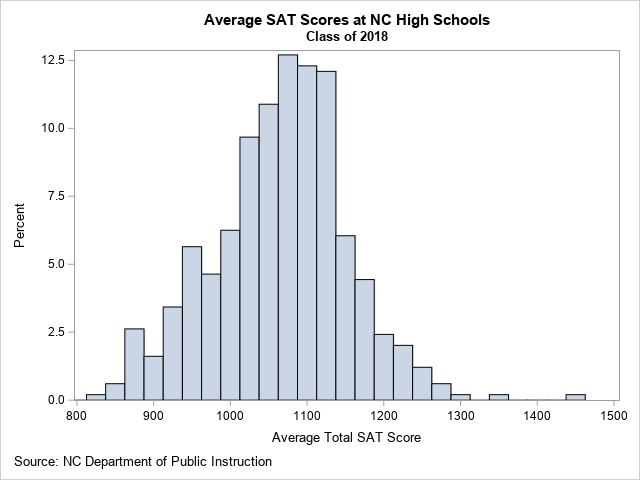From this graph, you can determine several facts about the data:

1. For most NC schools, the average SAT score is about 1100.
2. About 73% of NC schools have an average SAT score between 1000 and 1200.
3. There are a few schools that have much higher scores than the others. Those schools are Early College At Guilford (Total=1442), Raleigh Charter High School (Total=1356), and East Chapel Hill High (Total=1290).

Visualize the SAT math and English scores

If you want to compare the distributions of the math and English SAT scores, you can create a comparative histogram, as shown below: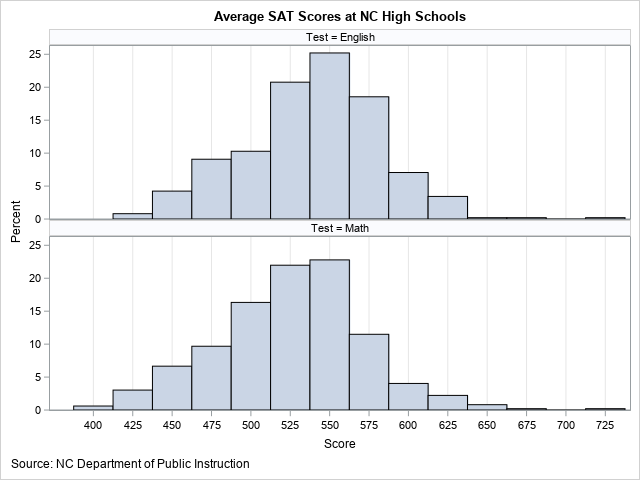In the comparative histogram, the average English scores for schools are shown in the top row; the math scores are in the bottom row. The English scores are generally higher, with a median value of 543. The median math score is about 15 points lower, with a value of 528.

Of course, the measurements in these two histograms are not independent. In reality, the math and English scores are paired, since every student takes both the math and English tests. Therefore, it makes sense to plot the joint distribution of the scores in a scatter plot, as follows: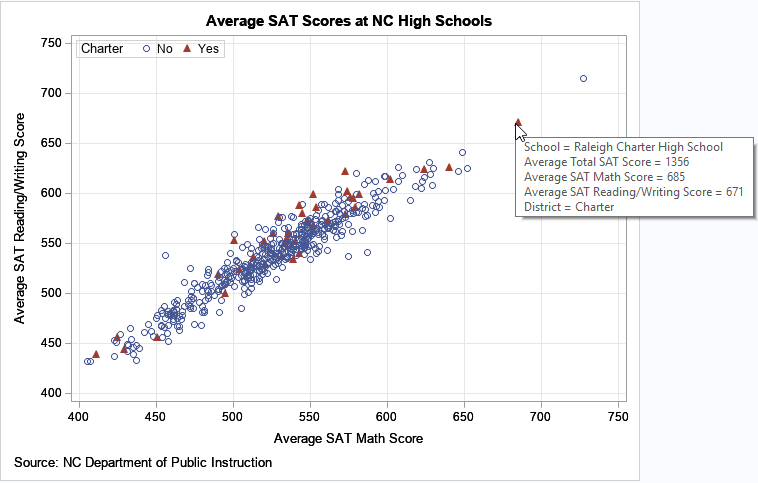In this plot, each school is represented by a marker. The math and English scores determine the placement of the marker. You can see that the math and English scores are highly correlated and linearly related. Schools with high (respectively, low) English scores tend to have high (respectively, low) math scores.

I used SAS to add tool tips to the graph. When I hover the cursor over a marker, the graph display information about the school and the average test scores. That technique makes it easy to identify the outliers. For example, the image above shows the tool-tip information for the Raleigh Charter High School, which has exceptionally high SAT scores.

And speaking of charter schools, legislators and school boards sometimes discuss the merits and disadvantages of using public tax dollars to establish and run charter schools. I have used red triangles to indicate the 39 charter schools in NC, which represent about 8% of the total schools. Overall, the distribution of SAT scores among the charter schools appears to be similar to the non-charter schools. I also created a comparative histogram of the charter/non-charter schools (not shown), which shows that the overall distribution is similar.

SAT scores for all NC public high schools

The previous graphs show the distribution of SAT scores for all public NC high schools, but do not enable you to easily compare different school districts. One way to compare school districts is to rank them according to the median scores for the schools within the district. You can create a graph that shows the school scores for each district. Because there are 115 school districts in NC, this graph will be very tall or wide. Nevertheless, it can be useful to rank the school districts and simultaneously see the distribution of scores for each school. The following plot displays the top 75 school districts, which collectively contain 385 high schools: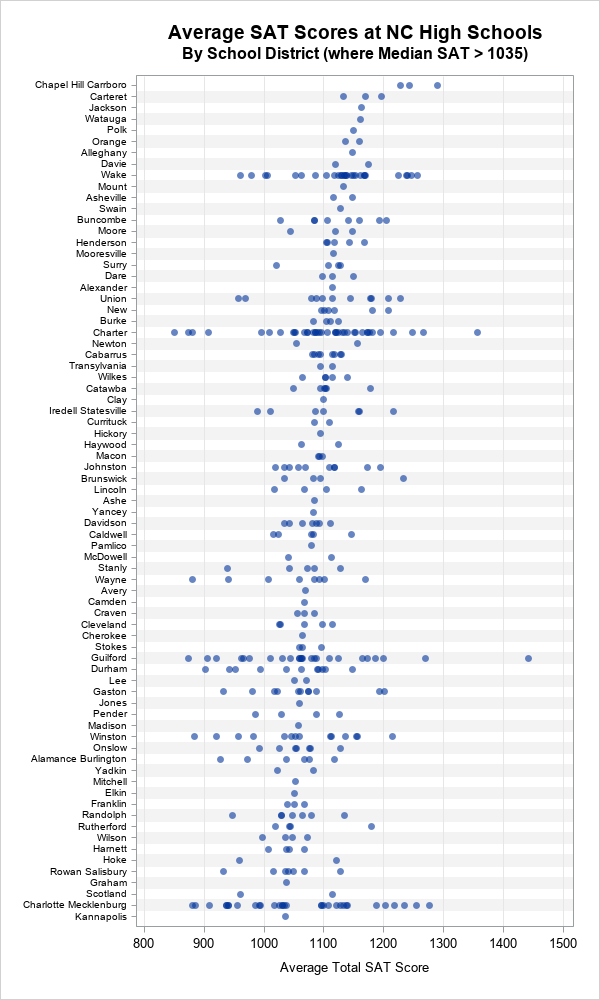The top school district is Chapel-Hill/Carrboro, which contains three high-performing high schools. The next few school districts are also small, having three or fewer high schools. Wake County, the second-largest school district in the state, is ninth in terms of the median SAT scores, but you can see considerable variation among the schools in the district. Other large school districts (Charter schools, Guilford, and Charlotte/Mecklenburg), show similar variation in scores.

Summary

By using data visualization, you can better understand the distribution of SAT scores across public high schools in NC. The graphs in this article enable you to see that there are several high-performing schools, lots of schools in the middle, and a few low-performing schools. Similarly, you can compare the test scores by school districts. The school-district graph enables you to see the variation of scores of schools within a district. Lastly, when you create these graphs in SAS, you can add tool tips that help you identify the individual schools within each school district.

In my next article, I will describe an alternative visualization that displays a box plot for each school district.

The post Visualize SAT scores in North Carolina appeared first on The DO Loop.Last year I published a series of blogs posts about how to create a calibration plot in SAS. A calibration plot is a way to assess the goodness of fit for a logistic model. It is a diagnostic graph that enables you to qualitatively compare a model's predicted probability of an event to the empirical probability. I am happy to report that in SAS/STAT 15.1 (SAS 9.4M6), you can create a calibration plot automatically by using the PLOTS=CALIBRATION option on the PROC LOGISTIC statement.

Calibration plots for a model of a binary response

To demonstrate how to create a calibration plot by using PROC LOGISTIC, consider the simulated data that I analyzed in "Calibration plots in SAS." The data contain a binary response variable, Y, which depends quadratically on a uniformly distributed explanatory variable, X. The following call to PROC LOGISTIC fits a quadratic the model to the data. The new GOF option requests an extensive set of goodness-of-fit statistics and the PLOTS=CALIBRATION option requests a calibration plot:

```/* NEW in SAS/STAT 15.1 (SAS 9.4M6): PLOTS=CALIBRATION option in PROC LOGISTIC */ title "Calibration Plot for a Quadratic Model"; title2 "Created by PROC LOGISTIC"; proc logistic data=LogiSim plots=calibration(CLM ShowObs); model y(Event='1') = x x*x / GOF; /* New in 15.1: More goodness-of-fit statistics */ run;```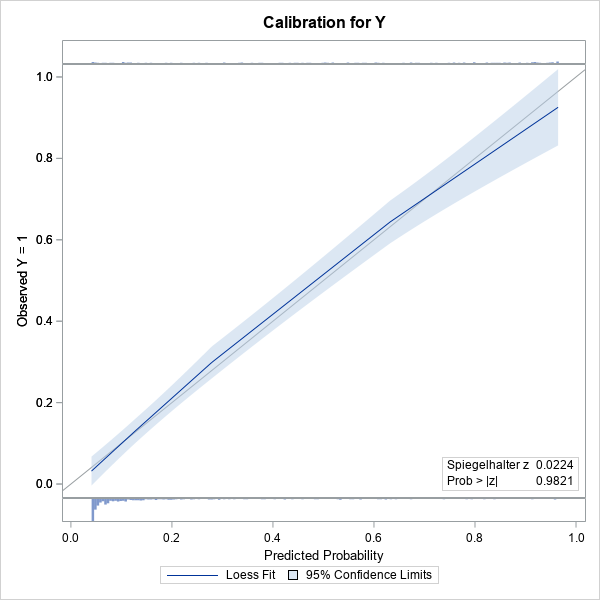The calibration plot is shown. (Click to enlarge.) The plot contains a gray diagonal line, which represents perfect calibration. If most of the predicted responses agree with the observed responses, then the blue curve should be close to the diagonal line. That is the case in this example. The light blue band is a 95% confidence region for the loess fit and is created by using the CLM option.

Because I used the SHOWOBS option, the calibration plot displays tiny histograms along the top and bottom of the plot. The histograms indicate the distribution of the Y=0 and Y=1 responses. The article "Use a fringe plot to visualize binary data in logistic models" explains more about how fringe plots can add insight to graphs that involve a binary response variable.

The lower right corner of the calibration plot contains one of the many goodness-of-fit statistics that are computed when you use the GOF option on the MODEL statement. A small p-value would indicate a lack of fit. In this case, there is no reason to suspect a lack of fit. The following table shows other goodness-of-fit tests. None of the p-values are small, so none of the tests indicate lack of fit.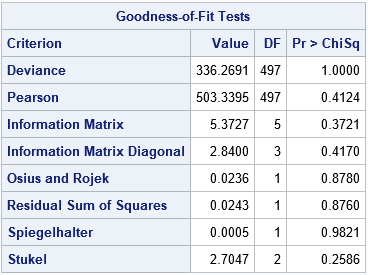Calibration plots for a polytomous response

An exciting feature of the calibration plots in PROC LOGISTIC is that you can use them for a polytomous response model. Derr (2013) ﬁts a proportional odds model that predicts the probability of the severity of black-lung disease from the length of exposure to coal dust in 371 coal miners. The response variable, Severity, has the levels 'Severe', 'Moderate', and 'Normal'. The following statement create the data and model and request calibration plots for the model.

```/* Data, from McCullagh and Nelder (1989, p. 179), used in Derr (2013, p. 8-10). The severity of pneumoconiosis (black lung disease) in coal miners and the number of years of exposure. */ data Coal; input Severity \$ @@; do i=1 to 8; input Exposure freq @@; log10Exposure=log10(Exposure); output; end; datalines; Normal 5.8 98 15 51 21.5 34 27.5 35 33.5 32 39.5 23 46 12 51.5 4 Moderate 5.8 0 15 2 21.5 6 27.5 5 33.5 10 39.5 7 46 6 51.5 2 Severe 5.8 0 15 1 21.5 3 27.5 8 33.5 9 39.5 8 46 10 51.5 5 ;   title 'Severity of Black Lung vs Log10(Years Exposure)'; proc logistic data=Coal rorder=data plots=Calibration(CLM); freq freq; model Severity(descending) = log10Exposure; effectplot / noobs individual; run;```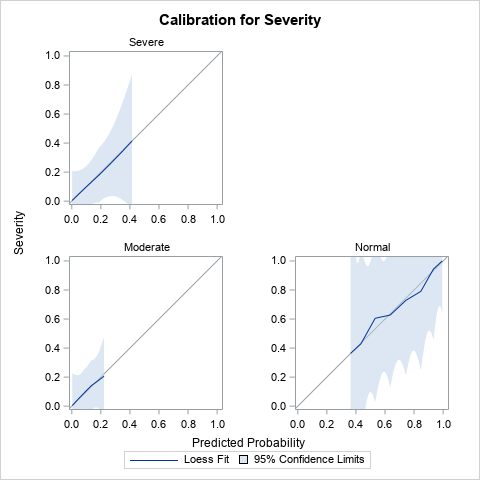Derr (2013) discusses the results of the analysis, which are not shown here. I've displayed only the calibration plot for the model. Notice that PROC LOGISTIC creates a panel of three calibration plots, one for each response level. The calibration curves all lie close to the diagonal, so the diagnostic plots do not indicate a lack of calibration for any part of the model.

Summary

In summary, the PLOTS=CALIBRATION option in SAS/STAT 15.1 enables you to automatically create a calibration plot. The calibration plot is a diagnostic plot that qualitatively compares a model's predicted and empirical probabilities. You can use the PLOTS=CALIBRATION option on the PROC LOGISTIC statement to create a calibration plot. The CALIBRATION option supports several suboptions, which you can read about in the documentation for the PROC LOGISTIC statement.

You can download the SAS code used in this article, which includes SAS code that demonstrates how to create a calibration plot manually.

The post An easier way to create a calibration plot in SAS appeared first on The DO Loop.Maybe if we think and wish and hope and pray
It might come true.
Oh, wouldn't it be nice?

The Beach Boys

Months ago, I wrote about how to use the EFFECT statement in SAS to perform regression with restricted cubic splines. This is the modern way to use splines in a regression analysis in SAS, and it replaces the need to use older macros such as Frank Harrell's %RCSPLINE macro. I shared my blog post with a colleague at SAS and mentioned that the process could be simplified. In order to specify the placement of the knots as suggested by Harrell (Regression Modeling Strategies, 2010 and 2015), I had to use PROC UNIVARIATE to get the percentiles of the explanatory variable. "Wouldn't it be nice," I said, "if the EFFECT statement could perform that computation automatically?"

I am happy to report that the 15.1 release of SAS/STAT (SAS 9.4M6) includes a new option that makes it easy to place internal knots at percentiles of the data. You can now use the KNOTMETHOD=PERCENTILELIST option on the EFFECT statement to place knots. For example, the following statement places five internal knots at percentiles that are recommended in Harrell's book:
EFFECT spl = spline(x / knotmethod=percentilelist(5 27.5 50 72.5 95));

An example of using restricted cubic in regression in SAS

Restricted cubic splines are also called "natural cubic splines." This section shows how to perform a regression fit by using restricted cubic splines in SAS.

For the example, I use the same Sashelp.Cars data that I used in the previous article. For clarity, the following SAS DATA step renames the Weight and MPG_City variables to X and Y, respectively. If you want to graph the regression curve, you can sort the data by the X variable, but this step is not required to perform the regression.

```/* create (X,Y) data from the Sashelp.Cars data. Sort by X for easy graphing. */ data Have; set sashelp.cars; rename mpg_city = Y weight = X model = ID; run;   proc sort data=Have; by X; run;```

The following call to PROC GLMSELECT includes an EFFECT statement that generates a natural cubic spline basis using internal knots placed at specified percentiles of the data. The MODEL statement fits the regression model and the OUTPUT statement writes an output data set that contains the predicted values. The SGPLOT procedure displays a graph of the regression curve overlaid on the data:

```/* fit data by using restricted cubic splines using SAS/STAT 15.1 (SAS 9.4M6) */ ods select ANOVA ParameterEstimates SplineKnots; proc glmselect data=Have; effect spl = spline(X/ details naturalcubic basis=tpf(noint) knotmethod=percentilelist(5 27.5 50 72.5 95); /* new in SAS/STAT 15.1 (SAS 9.4M6) */ model Y = spl / selection=none; /* fit model by using spline effects */ output out=SplineOut predicted=Fit; /* output predicted values */ quit;   title "Restricted Cubic Spline Regression"; title2 "Five Knots Placed at Percentiles"; proc sgplot data=SplineOut noautolegend; scatter x=X y=Y; series x=X y=Fit / lineattrs=(thickness=3 color=red); run;```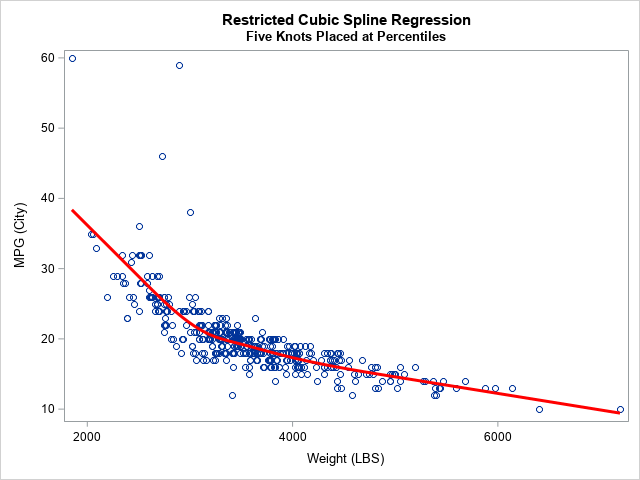In summary, the new KNOTMETHOD=PERCENTILELIST option on the EFFECT statement simplifies the process of using percentiles of a variable to place internal knots for a spline basis. The example shows knots placed at the 5th, 27.5th, 50th, 72.5th, and 95th percentiles of an explanatory variable. These heuristic values are recommended in Harrell's book. For more details about the EFFECT statement and how the location of knots affects the regression fit, see my previous article "Regression with restricted cubic splines in SAS."

You can download the complete SAS program that generates this example, which requires SAS/STAT 15.1 (SAS 9.4M6). If you have an earlier release of SAS, the program also shows how to perform the same computations by calling PROC UNIVARIATE to obtain the location of the knots.

The post An easier way to perform regression with restricted cubic splines in SAS appeared first on The DO Loop.Have you ever run a regression model in SAS but later realize that you forgot to specify an important option or run some statistical test? Or maybe you intended to generate a graph that visualizes the model, but you forgot? Years ago, your only option was to modify your program and rerun it. Current versions of SAS support a less painful alternative: you can use the STORE statement in many SAS/STAT procedures to save the model to an item store. You can then use the PLM procedure to perform many post-modeling analyses, including performing hypothesis tests, showing additional statistics, visualizing the model, and scoring the model on new data. This article shows four ways to use PROC PLM to obtain results from your regression model.

What is PROC PLM?

PROC PLM enables you to analyze a generalized linear model (or a generalized linear mixed model) long after you quit the SAS/STAT procedure that fits the model. PROC PLM was released with SAS 9.22 in 2010. This article emphasizes four features of PROC PLM:

• You can use the SCORE statement to score the model on new data.
• You can use the EFFECTPLOT statement to visualize the model.
• You can use the ESTIMATE, LSMEANS, SLICE, and TEST statements to estimate parameters and perform hypothesis tests.
• You can use the SHOW statement to display statistical tables such as parameter estimates and fit statistics.

For an introduction to PROC PLM, see "Introducing PROC PLM and Postfitting Analysis for Very General Linear Models" (Tobias and Cai, 2010). The documentation for the PLM procedure includes more information and examples.

To use PROC PLM you must first use the STORE statement in a regression procedure to create an item store that summarizes the model. The following procedures support the STORE statement: GEE, GENMOD, GLIMMIX, GLM, GLMSELECT, LIFEREG, LOGISTIC, MIXED, ORTHOREG, PHREG, PROBIT, SURVEYLOGISTIC, SURVEYPHREG, and SURVEYREG.

The example in this article uses PROC LOGISTIC to analyze data about pain management in elderly patients who have neuralgia. In the PROC LOGISTIC documentation, PROC LOGISTIC fits the model and performs all the post-fitting analyses and visualization. In the following program, PROC LOGIST fits the model and stores it to an item store named PainModel. In practice, you might want to store the model to a permanent libref (rather than WORK) so that you can access the model days or weeks later.

```Data Neuralgia; input Treatment \$ Sex \$ Age Duration Pain \$ @@; datalines; P F 68 1 No B M 74 16 No P F 67 30 No P M 66 26 Yes B F 67 28 No B F 77 16 No A F 71 12 No B F 72 50 No B F 76 9 Yes A M 71 17 Yes A F 63 27 No A F 69 18 Yes B F 66 12 No A M 62 42 No P F 64 1 Yes A F 64 17 No P M 74 4 No A F 72 25 No P M 70 1 Yes B M 66 19 No B M 59 29 No A F 64 30 No A M 70 28 No A M 69 1 No B F 78 1 No P M 83 1 Yes B F 69 42 No B M 75 30 Yes P M 77 29 Yes P F 79 20 Yes A M 70 12 No A F 69 12 No B F 65 14 No B M 70 1 No B M 67 23 No A M 76 25 Yes P M 78 12 Yes B M 77 1 Yes B F 69 24 No P M 66 4 Yes P F 65 29 No P M 60 26 Yes A M 78 15 Yes B M 75 21 Yes A F 67 11 No P F 72 27 No P F 70 13 Yes A M 75 6 Yes B F 65 7 No P F 68 27 Yes P M 68 11 Yes P M 67 17 Yes B M 70 22 No A M 65 15 No P F 67 1 Yes A M 67 10 No P F 72 11 Yes A F 74 1 No B M 80 21 Yes A F 69 3 No ;   title 'Logistic Model on Neuralgia'; proc logistic data=Neuralgia; class Sex Treatment; model Pain(Event='Yes')= Sex Age Duration Treatment; store PainModel / label='Neuralgia Study'; /* or use mylib.PaimModel for permanent storage */ run;```

The LOGISTIC procedure models the presence of pain based on a patient's medication (Drug A, Drug B, or placebo), gender, age, and duration of pain. After you fit the model and store it, you can use PROC PLM to perform all sorts of additional analyses, as shown in the subsequent sections.

Use PROC PLM to score new data

An important application of regression models is to predict the response variable for new data. The following DATA step defines three new patients. The first two are females who are taking Drug B. The third is a male who is taking Drug A:

```/* 1.Use PLM to score future obs */ data NewPatients; input Treatment \$ Sex \$ Age Duration; datalines; B F 63 5 B F 79 16 A M 74 12 ;   proc plm restore=PainModel; score data=NewPatients out=NewScore predicted LCLM UCLM / ilink; /* ILINK gives probabilities */ run;   proc print data=NewScore; run;```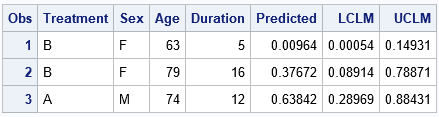The output shows the predicted pain level for the three patients. The younger woman is predicted to have a low probability (0.01) of pain. The model predicts a moderate probability of pain (0.38) for the older woman. The model predicts a 64% chance that the man will experience pain.

Notice that the PROC PLM statement does not use the original data. In fact, the procedure does not support a DATA= option but instead uses the RESTORE= option to read the item store. The PLM procedure cannot create plots or perform calculations that require the data because the data are not part of the item store.

Use PROC PLM to visualize the model

I've previously written about how to use the EFFECTPLOT statement to visualize regression models. The EFFECTPLOT statement has many options. However, because PROC PLM does not have access to the original data, the EFFECTPLOT statement in PROC PLM cannot add observations to the graphs.

Although the EFFECTPLOT statement is supported natively in the LOGISTIC and GENMOD procedure, it is not directly supported in other procedures such as GLM, MIXED, GLIMMIX, PHREG, or the SURVEY procedures. Nevertheless, because these procedures support the STORE statement, you can use the EFFECTPLOT statement in PROC PLM to visualize the models for these procedures. The following statement uses the EFFECTPLOT statement to visualize the probability of pain for female and male patients that are taking each drug treatment:

```/* 2. Use PROC PLM to create an effect plot */ proc plm restore=PainModel; effectplot slicefit(x=Age sliceby=Treatment plotby=Sex); run;```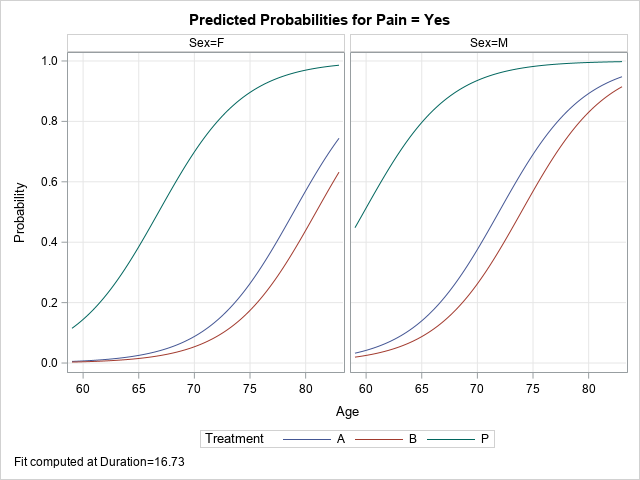The graphs summarize the model. For both men and women, the probability of pain increases with age. At a given age, the probability of pain is lower for the non-placebo treatments, and the probability is slightly lower for the patients who use Drug B as compared to Drug A. These plots are shown at the mean value of the Duration variable.

Use PROC PLM to compute contrasts and other estimates

One of the main purposes of PROC PLM Is to perform postfit estimates and hypothesis tests. The simplest is a pairwise comparison that estimates the difference between two levels of a classification variable. For example, in the previous graph the probability curves for the Drug A and Drug B patients are close to each other. Is there a significant difference between the two effects? The following ESTIMATE statement estimates the (B vs A) effect. The EXP option exponentiates the estimate so that you can interpret the 'Exponentiated' column as the odds ratio between the drug treatments. The CL option adds confidence limits for the estimate of the odds ratio. The odds ratio contains 1, so you cannot conclude that Drug B is significantly more effective that Drug A at reducing pain.

```/* 3. Use PROC PLM to create contrasts and estimates */ proc plm restore=PainModel; /* 'Exponentiated' column is odds ratio between treatments */ estimate 'Pairwise B vs A' Treatment 1 -1 / exp CL; run;```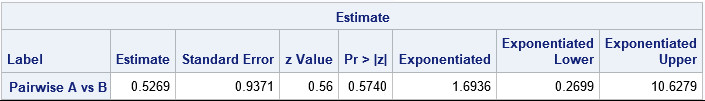Use PROC PLM to display statistics from the analysis

One of the more useful features of PROC PLM is that you can use the SHOW statement to display tables of statistics from the original analysis. If you want to see the ParameterEstimates table again, you can do that (SHOW PARAMETERS). You can even display statistics that you did not compute originally, such as an estimate of the covariance of the parameters (SHOW COVB). Lastly, if you have the item store but have forgotten what program you used to generate the model, you can display the program (SHOW PROGRAM). The following statements demonstrate the SHOW statement. The results are not shown.

```/* 4. Use PROC PLM to show statistics or the original program */ proc plm restore=PainModel; show Parameters COVB Program; run;```

Summary

In summary, the STORE statement in many SAS/STAT procedures enables you to store various regression models into an item store. You can use PROC PLM to perform additional postfit analyses on the model, including scoring new data, visualizing the model, hypothesis testing, and (re)displaying additional statistics. This technique is especially useful for long-running models, but it is also useful for confidential data because the data are not needed for the postfit analyses.

The post 4 reasons to use PROC PLM for linear regression models in SAS appeared first on The DO Loop.In machine learning and other model building techniques, it is common to partition a large data set into three segments: training, validation, and testing.

• Training data is used to fit each model.
• Validation data is a random sample that is used for model selection. These data are used to select a model from among candidates by balancing the tradeoff between model complexity (which fit the training data well) and generality (but they might not fit the validation data). These data are potentially used several times to build the final model
• Test data is a hold-out sample that is used to assess final model and estimate its prediction error. It is only used at the end of the model-building process.

I've seen many questions about how to use SAS to split data into training, validation, and testing data. (A common variation uses only training and validation.) There are basically two approaches to partitioning data:

• Specify the proportion of observations that you want in each role. For each observation, randomly assign it to one of the three roles. The number of observations assigned to each role will be a multinomial random variable with expected value N pk, where N is the number of observations and pk (k = 1, 2, 3) is the probability of assigning an observation to the k_th role. For this method, if you change the random number seed you will usually get a different number of observations each role because the size is a random variable.
• Specify the number of observations that you want in each role and randomly allocate that many observations.

This article uses the SAS DATA step to accomplish the first task and uses PROC SURVEYSELECT to accomplish the second. I also discuss how to split data into only two roles: training and validation.

It is worth mentioning that many model-selection routines in SAS enable you to split data by using the PARTITION statement. Example include the "SELECT" procedures (GLMSELECT, QUANTSELECT, HPGENSELECT...) and the ADAPTIVEREG procedure. However, be aware that the procedures might ignore observations that have missing values for the variables in the model.

Random partition into training, validation, and testing data

When you partition data into various roles, you can choose to add an indicator variable, or you can physically create three separate data sets. The following DATA step creates an indicator variable with values "Train", "Validate", and "Test". The specified proportions are 60% training, 30% validation, and 10% testing. You can change the values of the SAS macro variables to use your own proportions. The RAND("Table") function is an efficient way to generate the indicator variable.

```data Have; /* the data to partition */ set Sashelp.Heart; /* for example, use Heart data */ run;   /* If propTrain + propValid = 1, then no observation is assigned to testing */ %let propTrain = 0.6; /* proportion of trainging data */ %let propValid = 0.3; /* proportion of validation data */ %let propTest = %sysevalf(1 - &propTrain - &propValid); /* remaining are used for testing */   /* Randomly assign each observation to a role; _ROLE_ is indicator variable */ data RandOut; array p _temporary_ (&propTrain, &propValid); array labels \$ _temporary_ ("Train", "Validate", "Test"); set Have; call streaminit(123); /* set random number seed */ /* RAND("table") returns 1, 2, or 3 with specified probabilities */ _k = rand("Table", of p[*]); _ROLE_ = labels[_k]; /* use _ROLE_ = _k if you prefer numerical categories */ drop _k; run;   proc freq data=RandOut order=freq; tables _ROLE_ / nocum; run;```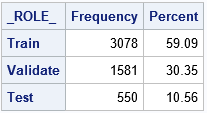A shown by the output of PROC FREQ, the proportion of observations in each role is approximately the same as the specified proportions. For this random number seed, the proportions are 59.1%, 30.4%, and 10.6%. If you change the random number seed, you will get a different assignment of observations to roles and also a different proportion of data in each role.

The observant reader will notice that there are only two elements in the array of probabilities (p) that is used in the RAND("Table") call. This is intentional. The documentation for the RAND("Table") function states that if the sum of the specified probabilities is less than 1, then the quantity 1 – Σ pi is used as the probability of the last event. By specifying only two values in the p array, the same program works for partitioning the data into two pieces (training and validation) or three pieces (and testing).

Create random training, validation, and testing data sets

Some practitioners choose to create three separate data sets instead of adding an indicator variable to the existing data. The computation is exactly the same, but you can use the OUTPUT statement to direct each observation to one of three output data sets, as follows:

```/* create a separate data set for each role */ data Train Validate Test; array p _temporary_ (&propTrain, &propValid); set Have; call streaminit(123); /* set random number seed */ /* RAND("table") returns 1, 2, or 3 with specified probabilities */ _k = rand("Table", of p[*]); if _k = 1 then output Train; else if _k = 2 then output Validate; else output Test; drop _k; run;```
```NOTE: The data set WORK.TRAIN has 3078 observations and 17 variables. NOTE: The data set WORK.VALIDATE has 1581 observations and 17 variables. NOTE: The data set WORK.TEST has 550 observations and 17 variables.```

This example uses the same random number seed as the previous example. Consequently, the three output data sets have the same observations as are indicated by the partition variable (_ROLE_) in the previous example.

Specify the number of observations in each role

Instead of specifying a proportion, you might want to specify the exact number of observations that are randomly assigned to each role. The advantage of this technique is that changing the random number seed does not change the number of observations in each role (although it does change which observations are assigned to each role). The SURVEYSELECT procedure supports the GROUPS= option, which you can use to specify the number of observations.

The GROUPS= option requires that you specify integer values. For this example, the original data contains 5209 observations but 60% of 5209 is not an integer. Therefore, the following DATA step computes the number of observations as ROUND(N p) for the training and validation sets. These integer values are put into macro variables and used in the GROUPS= option on the PROC SURVEYSELECT statement. You can, of course, skip the DATA step and specify your own values such as groups=(3200, 1600, 409).

```/* Specify the sizes of the train/validation/test data from proportions */ data _null_; if 0 then set sashelp.heart nobs=N; /* N = total number of obs */ nTrain = round(N * &propTrain); /* size of training data */ nValid = round(N * &propValid); /* size of validataion data */ call symputx("nTrain", nTrain); /* put integer into macro variable */ call symputx("nValid", nValid); call symputx("nTest", N - nTrain - nValid); run;   /* randomly assign observations to three groups */ proc surveyselect data=Have seed=12345 out=SSOut groups=(&nTrain, &nValid, &nTest); /* if no Test data, use GROUPS=(&nTrain, &nValid) */ run;   proc freq data=SSOut order=freq; tables GroupID / nocum; /* GroupID is name of indicator variable */ run;```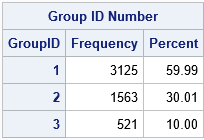The training, validation, and testing groups contain 3125, 1563, and 521 observations, respectively. These numbers are the closest integer approximations to 60%, 30% and 10% of the 5209 observations. Notice that the output from the SURVEYSELECT procedure uses the values 1, 2, and 3 for the GroupID indicator variable. You can use PROC FORMAT to associate those numbers with labels such as "Train", "Validate", and "Test".

In summary, there are two basic programming techniques for randomly partitioning data into training, validation, and testing roles. One way uses the SAS DATA step to randomly assign each observation to a role according to proportions that you specify. If you use this technique, the size of each group is random. The other way is to use PROC SURVEYSELECT to randomly assign observations to roles. If you use this technique, you must specify the number of observation in each group.

The post Create training, validation, and test data sets in SAS appeared first on The DO Loop.A quantile-quantile plot (Q-Q plot) is a graphical tool that compares a data distribution and a specified probability distribution. If the points in a Q-Q plot appear to fall on a straight line, that is evidence that the data can be approximately modeled by the target distribution. Although it is not necessary, some data analysts like to overlay a reference line to help "guide their eyes" as to whether the values in the plot fall on a straight line. This article describes three ways to overlay a reference line on a Q-Q plot. The first two lines are useful during the exploratory phase of data analysis; the third line visually represents the estimates of the location and scale parameters in the fitted model distribution. The three lines are:

• A line that connect the 25th and 75th percentiles of the data and reference distributions
• A least squares regression line
• A line whose intercept and slope are determined by maximum likelihood estimates of the location and scale parameters of the target distribution.

If you need to review Q-Q plots, see my previous article that describes what a Q-Q plot is, how to construct a Q-Q plot in SAS, and how to interpret a Q-Q plot.

Create a basic Q-Q plot in SAS

Let me be clear: It is not necessary to overlay a line on a Q-Q plot. You can display only the points on a Q-Q plot and, in fact, that is the default behavior in SAS when you create a Q-Q plot by using the QQPLOT statement in PROC UNIVARIATE.

The following DATA step generates 97 random values from an exponential distribution with shape parameter σ = 2 and three artificial "outliers." The call to PROC UNIVARIATE creates a Q-Q plot, which is shown:

```data Q(keep=y); call streaminit(321); do i = 1 to 97; y = round( rand("Expon", 2), 0.001); /* Y ~ Exp(2), rounded to nearest 0.001 */ output; end; do y = 10,11,15; output; end; /* add outliers */ run;   proc univariate data=Q; qqplot y / exp grid; /* plot data quantiles against Exp(1) */ ods select QQPlot; ods output QQPlot=QQPlot; /* for later use: save quantiles to a data set */ run;```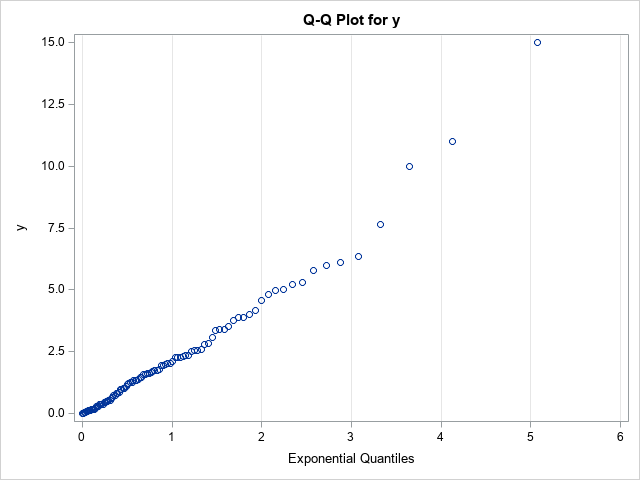The vertical axis of the Q-Q plot displays the sorted values of the data; the horizontal axis displays evenly spaced quantiles of the standardized target distribution, which in this case is the exponential distribution with scale parameter σ = 1. Most of the points appear to fall on a straight line, which indicates that these (simulated) data might be reasonably modeled by using an exponential distribution. The slope of the line appears to be approximately 2, which is a crude estimate of the scale parameter (σ). The Y-intercept of the line appears to be approximately 0, which is a crude estimate of the location parameter (the threshold parameter, θ).

Although the basic Q-Q plot provides all the information you need to decide that these data can be modeled by an exponential distribution, some data sets are less clear. The Q-Q plot might show a slight bend or wiggle, and you might want to overlay a reference line to assess how severely the pattern deviates from a straight line. The problem is, what line should you use?

A reference line for the Q-Q plot

Cleveland (Visualiizing Data, 1993, p. 31) recommends overlaying a line that connects the first and third quartiles. That is, let p25 and p75 be the 25th and 75th percentiles of the target distribution, respectively, and let y25 and y75 be the 25th and 75th percentiles of the ordered data values. Then Cleveland recommends plotting the line through the ordered pairs (p25, y25) and (p75, yy5).

In SAS, you can use PROC MEANS to compute the 25th and 75th percentiles for the X and Y variables in the Q-Q plot. You can then use the DATA step or PROC SQL to compute the slope of the line that passes between the percentiles. The following statements analyze the Q-Q plot data that was created by using the ODS OUTPUT statement in the previous section:

```proc means data=QQPlot P25 P75; var Quantile Data; /* ODS OUTPUT created the variables Quantile (X) and Data (Y) */ output out=Pctl P25= P75= / autoname; run;   data _null_; set Pctl; slope = (Data_P75 - Data_P25) / (Quantile_P75 - Quantile_P25); /* dy / dx */ /* if desired, put point-slope values into macro variables to help plot the line */ call symputx("x1", Quantile_P25); call symputx("y1", Data_P25); call symput("Slope", putn(slope,"BEST5.")); run;   title "Q-Q Plot with Reference Line"; title2 "Reference Line through First and Third Quartiles"; title3 "Slope = &slope"; proc sgplot data=QQPlot; scatter x=Quantile y=Data; lineparm x=&x1 y=&y1 slope=&slope / lineattrs=(color=Green) legendlabel="Percentile Estimate"; xaxis grid label="Exponential Quantiles"; yaxis grid; run;```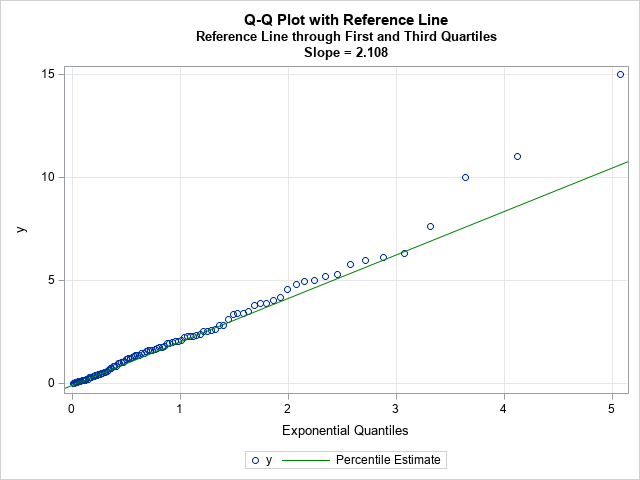Because the line passes through the first and third quartiles, the slope of the line is robust to outliers in the tails of the data. The line often provides a simple visual guide to help you determine whether the central portion of the data matches the quantiles of the specified probability distribution.

Keep in mind that this is a visual guide. The slope and intercept for this line should not be used as parameter estimates for the location and scale parameters of the probability distribution, although they could be used as an initial guess for an optimization that estimates the location and scale parameters for the model distribution.

Regression lines as visual guides for a Q-Q plot

Let's be honest, when a statistician sees a scatter plot for which the points appear to be linearly related, there is a Pavlovian reflex to fit a regression line to the values in the plot. However, I can think of several reasons to avoid adding a regression line to a Q-Q plot:

• The values in the Q-Q plot do not satisfy the assumptions of ordinary least squares (OLS) regression. For example, the points are not a random sample and there is no reason to assume that the errors in the Y direction are normally distributed.
• In practice, the tails of the probability distribution rarely match the tails of the data distribution. In fact, the points to the extreme left and right of a Q-Q plot often exhibit a systematic bend away from a straight line. In an OLS regression, these extreme points will be high-leverage points that will unduly affect the OLS fit.

If you choose to ignore these problems, you can use the REG statement in PROC SGPLOT to add a reference line. Alternatively, you can use PROC REG in SAS (perhaps with the NOINT option if the location parameter is zero) to obtain an estimate of the slope:

```proc reg data=QQPlot plots=NONE; model Data = Quantile / NOINT; /* use NOINT when location parameter is 0 */ ods select ParameterEstimates; quit;   title2 "Least Squares Reference Line"; proc sgplot data=QQPlot; scatter x=Quantile y=Data; lineparm x=0 y=0 slope=2.36558 / lineattrs=(color=Red) legendlabel="OLS Estimate"; xaxis grid label="Exponential Quantiles"; yaxis grid; run;```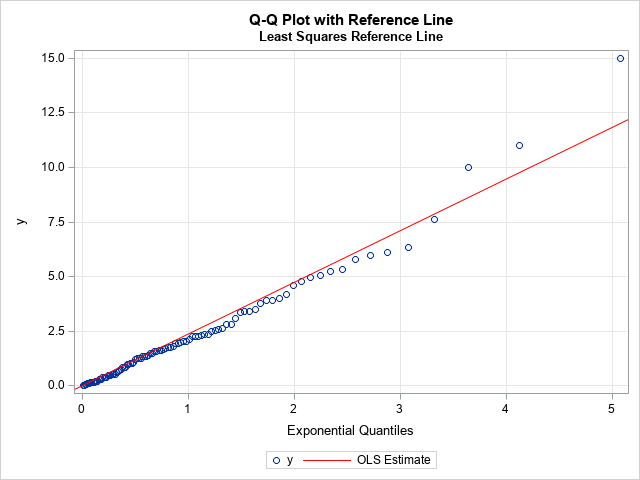For these data, I used the NOINT option to set the threshold parameter to 0. The zero-intercept line with slope 2.36558 is overlaid on the Q-Q plot. As expected, the outliers in the upper-right corner of the Q-Q plot have pulled the regression line upward, so the regression line has a steeper slope than the reference line based on the first and third quartiles. Because the tails of an empirical distribution often differ from the tails of the target distribution, the regression-based reference line can be misleading. I do not recommend its use.

Maximum likelihood estimates

The previous sections describe two ways to overlay a reference line during the exploratory phase of the data analysis. The purpose of the reference line is to guide your eye and help you determine whether the points in the Q-Q plot appear to fall on a straight line. If so, you can move to the modeling phase.

In the modeling phase, you use a parameter estimation method to fit the parameters in the target distribution. Maximum likelihood estimation (MLE) is often the method-of-choice for estimating parameters from data. You can use the HISTOGRAM statement in PROC UNIVARIATE to obtain a maximum likelihood estimate of the shape parameter for the exponential distribution, which turns out to be 2.21387. If you specify the location and scale parameters in the QQPLOT statement, PROC UNIVARIATE will automatically overlay a line that represents that fitted values:

```proc univariate data=Q; histogram y / exp; qqplot y / exp(threshold=0 scale=est) odstitle="Q-Q Plot with MLE Estimate" grid; ods select ParameterEstimates GoodnessOfFit QQPlot; run;```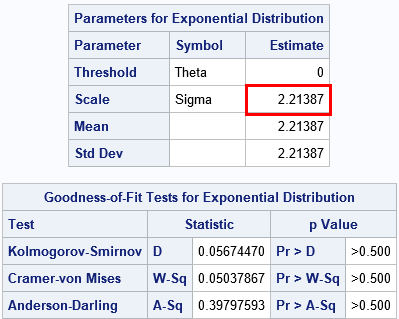The ParameterEstimates table shows the maximum likelihood estimate. The GoodnessOfFit table shows that there is no evidence to reject the hypothesis that these data came from an Exp(σ=2.21) distribution.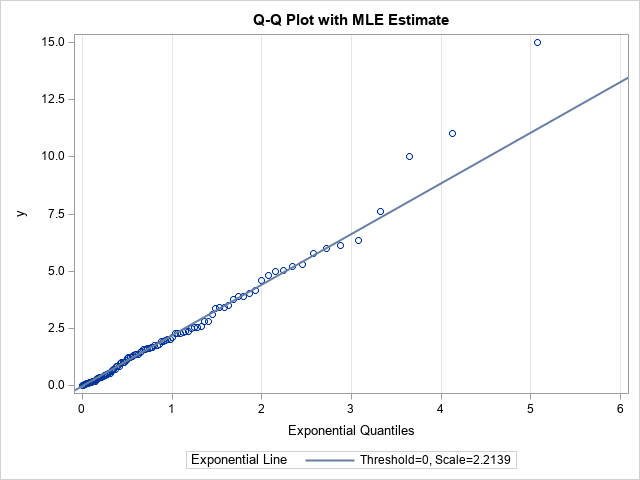Notice the distinction between this line and the previous lines. This line is the result of fitting the target distribution to the data (MLE) whereas the previous lines were visual guides. When you display a Q-Q plot that has a diagonal line, you should state how the line was computed.

In conclusion, you can display a Q-Q plot without adding any reference line. If you choose to overlay a line, there are three common methods. During the exploratory phase of analysis, you can display a line that connects the 25th and 75th percentiles of the data and target distributions. (Some practitioners use an OLS regression line, but I do not recommend it.) During the modeling phase, you can use maximum likelihood estimation or some other fitting method to estimate the location and scale of the target distribution. Those estimates can be used as the intercept and slope, respectively, of a line on the Q-Q plot. PROC UNIVARIATE in SAS displays this line automatically when you fit a distribution.

The post Three ways to add a line to a Q-Q plot appeared first on The DO Loop.When you overlay two series in PROC SGPLOT, you can either plot both series on the same axis or you can assign one series to the main axis (Y) and another to a secondary axis (Y2). If you use the Y and Y2 axes, they are scaled independently by default, which is usually what you want. However, if the measurements for the two series are linearly related to each other, then you might want to specify the tick values for the Y2 axis so that they align with the corresponding tick marks for the Y axis. This article shows how to align the Y and Y2 axes in PROC SGPLOT in SAS for two common situations.

Different scales for one set of measurements

The simplest situation is a single set of data that you want to display in two different units. For example, you might use one axis to display the data in imperial units (pounds, gallons, degrees Fahrenheit, etc.) and the other axis to display the data in metric units (kilograms, liters, degrees Celsius, etc.).

To plot the data, define one variable for each unit. For example, the Sashelp.Class data records the weight for 19 students in pounds. The following DATA view creates a new variable that records the same data in kilograms. The subsequent call to PROC SGPLOT plots the pounds on the Y axis (left axis) and the kilograms on the Y2 axis (right axis). However, as you will see, there is a problem with the default scaling of the two axes:

```data PoundsKilos / view=PoundsKilos; set Sashelp.Class(rename=(Weight=Pounds)); Kilograms = 0.453592 * Pounds; /* convert pounds to kilos */ run;   title "Independent Axes"; title2 "Markers Do Not Align Correctly!"; /* the tick marks on each axis are independent */ proc sgplot data=PoundsKilos; scatter x=Height y=Pounds; scatter x=Height y=Kilograms / Y2Axis; run;```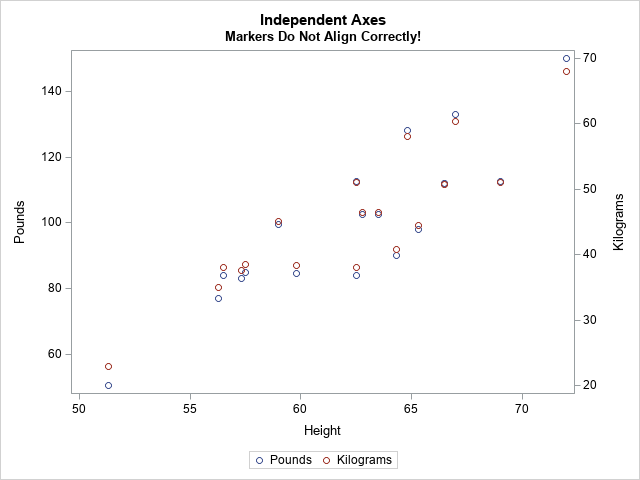The markers for the kilogram measurements should exactly overlap the markers for pounds, but they don't. The Y and Y2 axes are independently scaled because PROC SGPLOT does not know that pounds and kilograms are linearly related. The SGPLOT procedure displays each variable by using a range of round numbers (multiples of 10 or 20). The range for the Y2 axis is [20, 70] kilograms, which corresponds to a range of [44.1, 154.3] pounds. However, the range for the Y axis is approximately [50, 150] pounds. Because the axes display different ranges, the markers do not overlap.

To improve this graph, use the VALUES= and VALUESDISPLAY= options on the YAXIS statement (or Y2AXIS statement) to force the ticks marks on one axis to align with the corresponding tick marks on the other axis. In the following DATA step, I use the kilogram scale as the standard and compute the corresponding pounds.

```data Ticks; do Kilograms = 20 to 70 by 10; /* for each Y2 tick */ Pounds = Kilograms / 0.453592; /* convert kilos to pounds */ Approx = round(Pounds, 0.1); /* use rounded values to display tick values */ output; end; run; proc print; run;```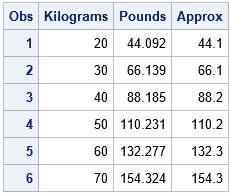You can use the Pounds column in the table to set the VALUES= list on the YAXIS statement. You can use the Approx column to set the VALUESDISPLAY= list, as follows:

```/* align tick marks on each axis */ title "Both Axes Use the Same Scale"; proc sgplot data=PoundsKilos noautolegend; scatter x=Height y=Pounds; /* Make sure the plots overlay exactly! Then you can set SIZE=0 */ scatter x=Height y=Kilograms / markerattrs=(size=0) Y2Axis; yaxis grid values=(44.092 66.139 88.185 110.231 132.277 154.324) valuesdisplay=('44.1' '66.1' '88.2' '110.2' '132.3' '154.3'); run;```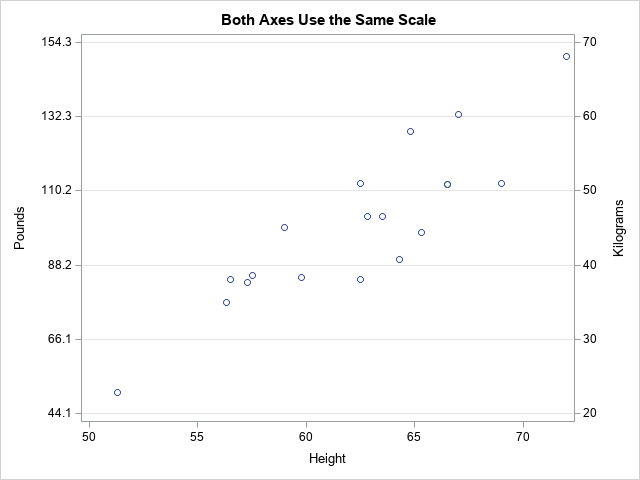Success! The markers for the two variables align exactly. After verifying that they align, you can use the MARKERATTRS=(SIZE=0) option to suppress the display of one of the markers.

Notice that the Y axis (pounds) no longer displays "nice numbers" because I put the tick marks at the same vertical heights on both axes. A different way to solve the misalignment problem is to use the MIN=, MAX=, THRESHOLDMIN=, and THRESHOLDMAX= options on both axes. This will enable both axes to use "nice numbers" while still aligning the data. If you want to try this approach, here are the YAXIS and Y2AXIS statements:

``` /* set the axes ranges to coresponding values */ yaxis grid thresholdmin=0 thresholdmax=0 min=44.1 max=154.3; y2axis grid thresholdmin=0 thresholdmax=0 min=20 max=70;```

Different scales for different measurements

Another situation that requires two Y axes is the case of two series that use different units. For example, you might want to plot the revenue for a US company (in dollars) and the revenue for a Japanese company (in yen) for a certain time period. You can use the conversion rate between yen and dollars to align the values on the axes. Of course, the conversion from Japanese yen to the US dollars changes each day, but you can use an average conversion rate to set the correspondence between the axes.

This situation also occurs when two devices use different methods to measure the same quantity. The following example shows measurements for a patient who receives a certain treatment. The quantity of a substance in the patient's blood is measured at baseline and for every hour thereafter. The quantity is measured in two ways: by using a traditional blood test and by using a new noninvasive device that measures electrical impedance. The following statements define and plot the data. The two axes are scaled by using the default method:

```data BloodTest1; label t="Hours after Medication" x="micrograms per deciliter" y="kiloOhms"; input x y @@; t = _N_ - 1; datalines; 169.0 45.5 130.8 33.4 109.0 23.8 94.1 19.8 86.3 20.4 78.4 18.7 76.1 16.1 72.2 16.7 70.0 11.9 69.8 14.6 69.5 10.6 68.7 12.7 67.3 16.9 ;   title "Overlay Measurements for Two Medical Devices"; title2 "Default Scaling"; proc sgplot data=BloodTest1; series x=t y=x / markers legendlabel="Standard Lab Value"; series x=t y=y / markers Y2Axis legendlabel="New Device"; xaxis values=(0 to 12 by 2); yaxis grid label="micrograms per deciliter"; y2axis grid label="kiloOhms"; run;```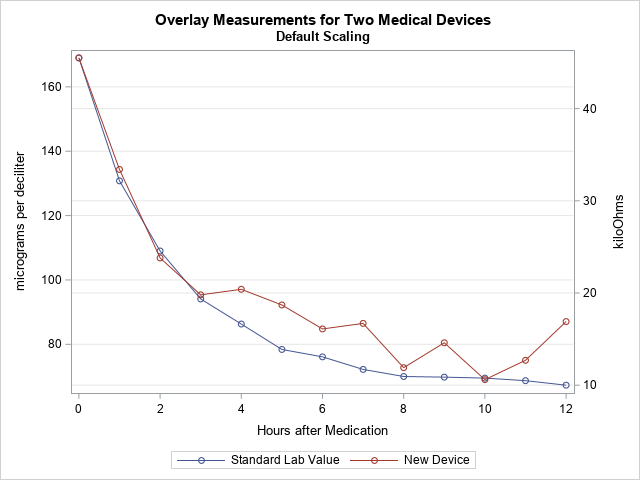In this graph, the Y axes are scaled independently. However, the company that manufactures the device used Deming regression to establish that the measurements from the two devices are linearly related by the equation Y = –10.56415 + 0.354463*X, where X is the measurement from the blood test. You can use this linear equation to set the scales for the two axes.

The following DATA step uses the Deming regression estimates to convert the tick marks on the Y axis into values for the Y2 axis. (Click here for the PROC PRINT output.) The call to PROC SGPLOT creates a graph in which the Y2 axis is aligned with the Y axis according to the Deming regression estimates.

```data Ticks; do Y1 = 60 to 160 by 20; /* use Deming regression to find one set of ticks in terms of the other */ Y2 = -10.56415 + 0.354463 * Y1; /* kiloOhms as a function of micrograms/dL */ Approx = round(Y2, 0.1); output; end; run;   proc print; run;   title "Align Y Axes for Different Series"; title2 "Measurements are Linearly Related"; proc sgplot data=BloodTest1; series x=t y=x / markers legendlabel="Standard Lab Value"; series x=t y=y / markers Y2Axis legendlabel="New Device"; xaxis values=(0 to 12 by 2); yaxis grid label="micrograms per deciliter" offsetmax=0.1 values=(60 to 160 by 20); /* the same offsets must be used in both YAXIS and Y2AXIS stmts */ y2axis grid label="kiloOhms" offsetmax=0.1 values=(10.7036 17.7929 24.8822 31.9714 39.0607 46.1499) valuesdisplay=('10.7' '17.8' '24.9' '32.0' '39.1' '46.1'); run;```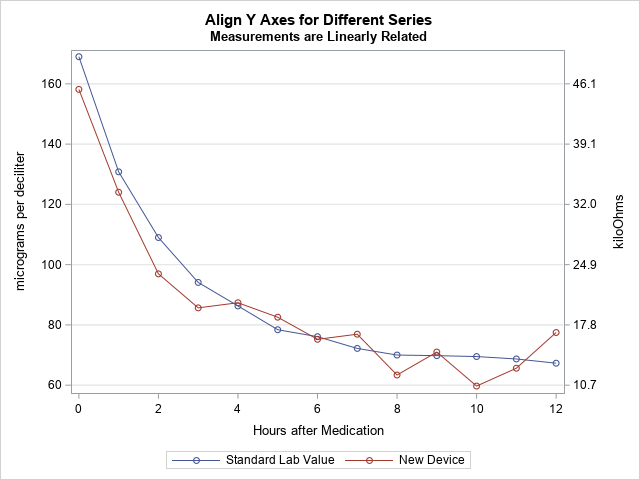In this new graph, the measurements are displayed on compatible scales and the reference lines connect round numbers on one axis to the corresponding values on the other axis.

The post How to align the Y and Y2 axes in PROC SGPLOT appeared first on The DO Loop.Numbers don't lie, but sometimes they don't reveal the full story. Last week I wrote about the most popular articles from The DO Loop in 2018. The popular articles are inevitably about elementary topics in SAS programming or statistics because those topics have broad appeal. However, I also write about advanced topics, which are less popular but fill an important niche in the SAS community. Not everyone needs to know how to fit a Pareto distribution in SAS or how to compute distance-based measures of correlation in SAS. Nevertheless, these topics are interesting to think about.

I believe that learning should not stop when we leave school. If you, too, are a lifelong learner, the following topics deserve a second look. I've included articles from four different categories.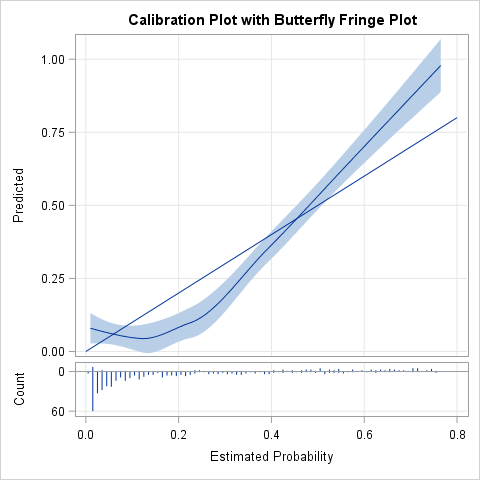Random numbers and resampling methods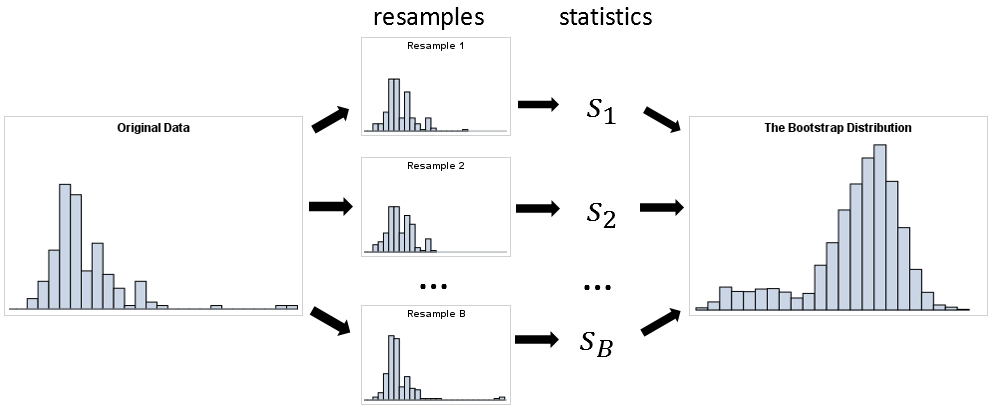Optimization

These articles are technical but provide tips and techniques that you might find useful. Choose a few topics that are unfamiliar and teach yourself something new in this New Year!

Do you have a favorite article from 2018 that I did not include on the list? Share it in a comment!

The post 10 posts from 2018 that deserve a second look appeared first on The DO Loop.Deming regression (also called errors-in-variables regression) is a total regression method that fits a regression line when the measurements of both the explanatory variable (X) and the response variable (Y) are assumed to be subject to normally distributed errors. Recall that in ordinary least squares regression, the explanatory variable (X) is assumed to be measured without error. Deming regression is explained in a Wikipedia article and in a paper by K. Linnet (1993).

A situation in which both X and Y are measured with errors arises when comparing measurements from different instruments or medical devices. For example, suppose a lab test measures the amount of some substance in a patient's blood. If you want to monitor this substance at regular intervals (for example, hourly), it is expensive, painful, and inconvenient to take the patient's blood multiple times. If someone invents a medical device that goes on the patient's finger and measures the substance indirectly (perhaps by measuring an electrical property such as bioimpedance), then that device would be an improved way to monitor the patient. However, as explained in Deal, Pate, and El Rouby (2009), the FDA would first need to approve the device and determine that it measures the response as accurately as the existing lab test. The FDA encourages the use of Deming regression for method-comparison studies.

Deming regression in SAS

There are several ways to compute a Deming Regression in SAS. The SAS FASTats site suggests maximum likelihood estimation (MLE) by using PROC OPTMODEL, PROC IML, or PROC NLMIXED. However, you can solve the MLE equations explicitly to obtain an explicit formula for the regression estimates. Deal, Pate, and El Rouby (2009) present a rather complicated macro, whereas Njoya and Hemyari (2017) use simple SQL statements. Both authors also provide SAS code for estimating the variance of the Deming regression estimates, either by using the jackknife method or by using the bootstrap. However, the resampling schemes in both papers are inefficient because they use a macro loop to perform the jackknife or bootstrap.

The following SAS DATA Step defines pairs of hypothetical measurements for 65 patients, each of whom received the standard lab test (measured in micrograms per deciliter) and the new noninvasive device (measured in kiloohms):

```data BloodTest; label x="micrograms per deciliter" y="kiloOhms"; input x y @@; datalines; 169.0 45.5 130.8 33.4 109.0 23.8 94.1 19.8 86.3 20.4 78.4 18.7 76.1 16.1 72.2 16.7 70.0 11.9 69.8 14.6 69.5 10.6 68.7 12.7 67.3 16.9 174.7 57.8 137.9 39.0 114.6 30.4 99.8 21.1 90.1 21.7 85.1 25.2 80.7 20.6 78.1 19.3 77.8 20.9 76.0 18.2 77.8 18.3 74.2 15.7 73.1 13.9 182.5 55.5 144.0 38.7 123.8 35.1 107.6 30.6 96.9 25.7 92.8 19.2 87.2 22.4 86.3 18.4 84.4 20.7 83.7 20.6 83.3 20.0 83.9 18.8 82.7 21.8 160.8 49.9 122.7 32.2 102.6 19.2 86.6 14.7 76.1 16.6 69.6 18.8 66.7 7.4 64.4 8.2 63.0 15.5 61.7 13.7 61.2 9.2 62.4 12.0 58.4 15.2 171.3 48.7 136.3 36.1 111.9 28.6 96.5 21.8 90.3 25.6 82.9 16.8 78.1 14.1 76.5 14.2 73.5 11.9 74.4 17.7 73.9 17.6 71.9 10.2 72.0 15.6 ;   title "Deming Regression"; title2 "Gold Standard (X) vs New Method (Y)"; proc sgplot data=BloodTest noautolegend; scatter x=x y=y; lineparm x=0 y=-10.56415 slope=0.354463 / clip; /* Deming regression estimates */ xaxis grid label="Lab Test (micrograms per deciliter)"; yaxis grid label="New Device (kiloohms)"; run;```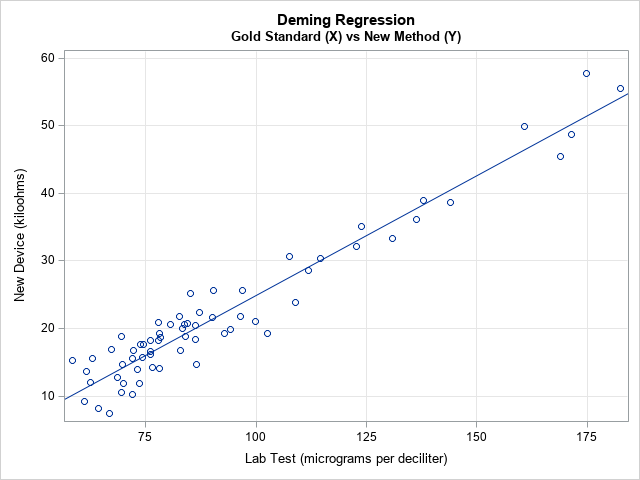The scatter plot shows the pairs of measurements for each patient. The linear pattern indicates that the new device is well calibrated with the standard lab test over a range of clinical values. The diagonal line represents the Deming regression estimate, which enables you to convert one measurement into another. For example, a lab test that reads 100 micrograms per deciliter is expected to correspond to 25 kiloohms on the new device and vice versa. (If you want to convert the new readings into the old, you can regress X onto Y and plot X on the vertical axis.)

The following SAS/IML function implements the explicit formulas that compute the slope and intercept of the Deming regression line:

```/* Deming Regression in SAS */ proc iml; start Deming(XY, lambda=); /* Equations from https://en.wikipedia.org/wiki/Deming_regression */ m = mean(XY); xMean = m; yMean = m; S = cov(XY); Sxx = S[1,1]; Sxy = S[1,2]; Syy = S[2,2]; /* if lambda is specified (eg, lambda=1), use it. Otherwise, estimate. */ if IsEmpty(lambda) then delta = Sxx / Syy; /* estimate of ratio of variance */ else delta = lambda; c = Syy - delta*Sxx; b1 = (c + sqrt(c**2 + 4*delta*Sxy**2)) / (2*Sxy); b0 = yMean - b1*xMean; return (b0 || b1); finish;   /* Test the program on the blood test data */ use BloodTest; read all var {x y} into XY; close; b = Deming(XY); print b[c={'Intercept' 'Slope'} L="Deming Regression"];```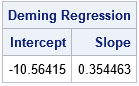The SAS/IML function can estimate the ratio of the variances of the X and Y variable. In the SAS macros by Deal, Pate, and El Rouby (2009) and Njoya and Hemyari (2017), the ratio is a parameter that is determined by the user. The examples in both papers use a ratio of 1, which assumes that the devices have an equal accuracy and use the same units of measurement. In the current example, the lab test and the electrical device use different units. The ratio of the variances for these hypothetical devices is about 7.4.

Standard errors of estimates

You might wonder how accurate the parameter estimates are. Linnet (1993) recommends using the jackknife method to answer that question. I have previously explained how to jackknife estimates in SAS/IML, and the following program is copied from that article:

```/* Helper modules for jackknife estimates of standard error and CI for parameters: */ /* return the vector {1,2,...,i-1, i+1,...,n}, which excludes the scalar value i */ start SeqExclude(n,i); if i=1 then return 2:n; if i=n then return 1:n-1; return (1:i-1) || (i+1:n); finish;   /* return the i_th jackknife sample for (n x p) matrix X */ start JackSamp(X,i); return X[ SeqExclude(nrow(X), i), ]; /* return data without i_th row */ finish;   /* 1. Compute T = statistic on original data */ T = b;   /* 2. Compute statistic on each leave-one-out jackknife sample */ n = nrow(XY); T_LOO = j(n,2,.); /* LOO = "Leave One Out" */ do i = 1 to n; J = JackSamp(XY,i); T_LOO[i,] = Deming(J); end;   /* 3. compute mean of the LOO statistics */ T_Avg = mean( T_LOO );   /* 4. Compute jackknife estimates of standard error and CI */ stdErrJack = sqrt( (n-1)/n * (T_LOO - T_Avg)[##,] ); alpha = 0.05; tinv = quantile("T", 1-alpha/2, n-2); /* use df=n-2 b/c both x and y are estimated */ Lower = T - tinv#stdErrJack; Upper = T + tinv#stdErrJack; result = T` || T_Avg` || stdErrJack` || Lower` || Upper`; print result[c={"Estimate" "Mean Jackknife Estimate" "Std Error" "Lower 95% CL" "Upper 95% CL"} r={'Intercept' 'Slope'}];```The formulas for the jackknife computation differs slightly from the SAS macro by Deal, Pate, and El Rouby (2009). Because both X and Y have errors, the t quantile must be computed by using n–2 degrees of freedom, not n–1.

If X and Y are measured on the same scale, then the methods are well-calibrated when the 95% confidence interval (CI) for the intercept includes 0 and the CI for the intercept includes 1. In this example, the devices use different scales. The Deming regression line enables you to convert from one measurement scale to the other; the small standard errors (narrow CIs) indicate that this conversion is accurate.

In summary, you can use a simple set of formulas to implement Deming regression in SAS. This article uses SAS/IML to implement the regression estimates and the jackknife estimate of the standard errors. You can also use the macros that are mentioned in the section "Deming regression in SAS," but the macros are less efficient, and you need to specify the ratio of the variances of the data vectors.

The post Deming regression for comparing different measurement methods appeared first on The DO Loop.Last year, I wrote more than 100 posts for The DO Loop blog. Of these, the most popular articles were about data visualization, SAS programming tips, and statistical data analysis. Here are the most popular articles from 2018 in each category.

Data Visualization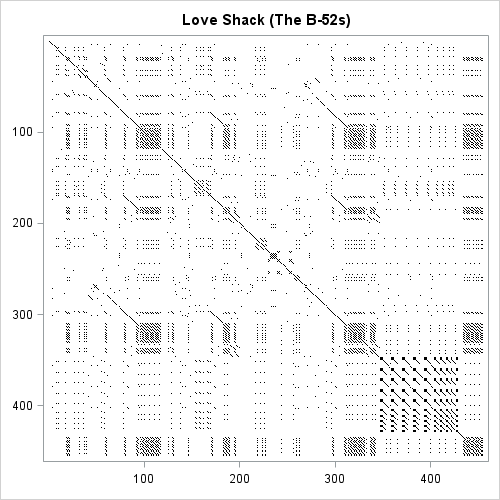Statistics and Data Analysis

I write this blog because I love to learn new things and share what I know with others. If you want to learn something new, read (or re-read!) these popular articles from 2018. Then share this page with one of your colleagues. Happy New Year! I hope we both have many opportunities to learn and share in 2019!

The post Top posts from <em>The DO Loop</em> in 2018 appeared first on The DO Loop.